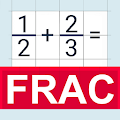# Fraction calculator Free APK

## andrei.brusentcov.fractioncalculator.free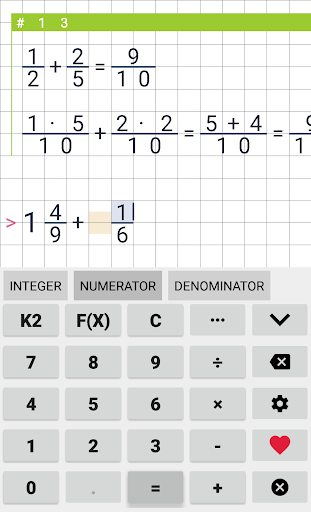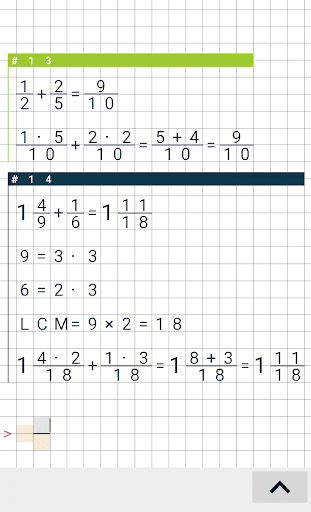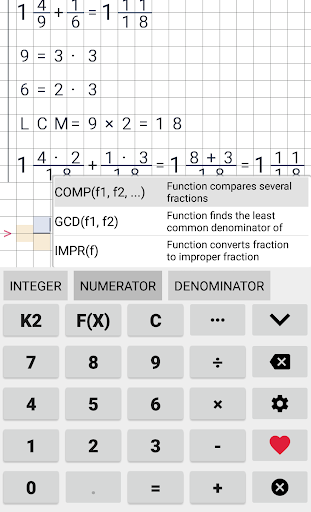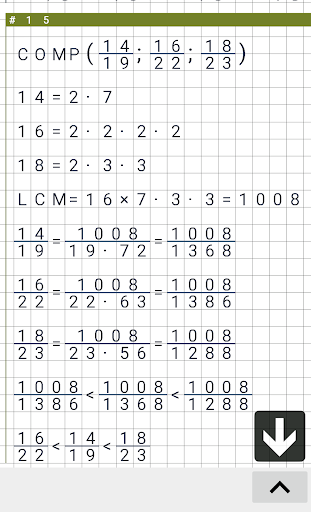Fractions calculator perform basic and advanced operations with fractions. Also shows detailed step-by-step information about fraction calculation procedure. This calculator supports adding, subtracting, dividing and multiplying fractions and returns an answer as a reduced fraction and a mixed number if it exists. It can also convert mixed numbers to fractions and vice versa. "Fraction calculator" makes math homework easier. Programm supports some advanced features including comparing fractions with different numerators and denominators.

Features:
– operations history stores up to 1000 entries. You can always see yesterday's calculations
– fractions multiplication
– fractions division
– comfortable keyboard
– comparing fractions
– fractions subtraction